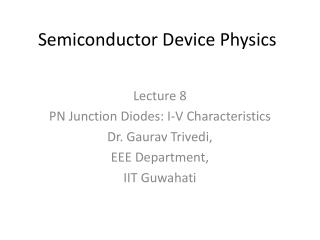DownloadDownload PresentationSemiconductor Device Physics

# Semiconductor Device Physics

Download Presentation## Semiconductor Device Physics

- - - - - - - - - - - - - - - - - - - - - - - - - - - E N D - - - - - - - - - - - - - - - - - - - - - - - - - - -
##### Presentation Transcript

1. Semiconductor Device Physics Lecture 8 PN Junction Diodes: I-V Characteristics Dr. GauravTrivedi, EEE Department, IIT Guwahati

2. Dominant breakdown mechanism is tunneling Empirical Observations of VBR • VBR decreases with increasing N, • VBR decreases with decreasing EG. • VBR : breakdown voltage

3. Breakdown Voltage, VBR

4. Breakdown Mechanism: Avalanching

5. Breakdown Mechanism: Zener Process

6. Effect of R–G in Depletion Region

7. Effect of R–G in Depletion Region

8. Effect of R–G in Depletion Region

9. Effect of R–G in Depletion Region

10. Effect of Series Resistance

11. Effect of High-Level Injection

12. High-Level Injection Effect

13. Summary

14. Minority-Carrier Charge Storage

15. Charge Control Approach

16. Charge Control Approach • Integrating over the n quasineutral region (after all terms multiplied byAdx), QP QP • Furthermore, in a p+n junction, 0 • So: In steady state

17. Charge Control Approach • In steady state, we can calculate pn junction current in two ways: • From slopes of Δnp(–xp) and Δpn(xn) • From steady-state charges QN and QP stored in each “excess minority charge distribution” • Therefore, • Similarly,

18. Charge Control Approach • Moreover, in a p+n junction: In steady state

19. Narrow-Base Diode • Narrow-base diode: a diode where the width of the quasineutral region on the lightly doped side of the junction is on the order of or less than one diffusion length. n-side contact

20. Narrow-Base Diode I–V • We have the following boundary conditions: • Then, the solution is of the form: • Applying the boundary conditions, we have:

21. Narrow-Base Diode I–V • Solving for A1 and A2, and substituting back: • Note that • The solution can be written more compactly as

22. Narrow-Base Diode I–V • With decrease base width, xc’0: • Δpn is a linear function of x due to negligible thermal R–G in region much shorter than one diffusion length •  JPis constant • This approximation can be derived using Taylor series approximation

23. Narrow-Base Diode I–V • Because , then • Then, for a p+n junction:

24. Narrow-Base Diode I–V • If xc’ << LP, • Resulting • Increase of reverse bias means • Increase of reverse current • Increase of depletion width • Decrease of quasineutral region xc’=xc–xn

25. Wide-Base Diode • Rewriting the general solution for carrier excess, • For the case of wide-base diode (xc’>> LP), Back to ideal diode solution

26. Wide-Base Diode • Rewriting the general solution for diffusion current, • For the case of wide-base diode (xc’>> LP), Back to ideal diode solution

27. Small-Signal Diode Biasing • When reversed-biased, a pn junction diode becomes functionally equivalent to a capacitor, whose capacitance decreases as the reverse bias increases. • Biasing additional a.c. signal va can be viewed as a small oscillation of the depletion width about the steady state value. V0 << VA RS : serial resistance C : capacitance G : conductance Y : admittance

28. Total pnJunction Capacitance Junction / depletion capacitance, due to variation of depletion charges Minority carrier lifetime Diffusion capacitance, due to variation of stored minority charges in the quasineutral regions • CJ dominates at low forward biases, reverse biases. • CD dominates at moderate to high forward biases.

29. Relation Between CJ and VA • For asymmetrical step junction, NB : bulk semiconductor doping, NA or ND as appropriate. • Therefore,